International
Tables for
Crystallography
Volume D
Physical properties of crystals
Edited by A. Authier

International Tables for Crystallography (2006). Vol. D, ch. 1.1, pp. 12-14

## Section 1.1.4.5. Intrinsic symmetry of tensors

A. Authiera*

aInstitut de Minéralogie et de la Physique des Milieux Condensés, Bâtiment 7, 140 rue de Lourmel, 75015 Paris, France
Correspondence e-mail: aauthier@wanadoo.fr

| top | pdf |

#### 1.1.4.5.1. Introduction

| top | pdf |

The symmetry of a tensor representing a physical property or a physical quantity may be due either to its own nature or to the symmetry of the medium. The former case is called intrinsic symmetry. It is a property that can be exhibited both by physical property tensors or by field tensors. The latter case is the consequence of Neumann's principle and will be discussed in Section 1.1.4.6. It applies to physical property tensors.

| top | pdf |

#### 1.1.4.5.2.1. Tensors of rank 2

| top | pdf |

A bilinear form is symmetric if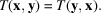Its components satisfy the relations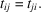The associated matrix, T, is therefore equal to its transpose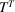: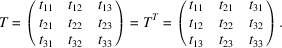In a space with n dimensions, the number of independent components is equal to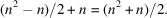#### Examples

 (1) The metric tensor (Section 1.1.2.2) is symmetric because the scalar product is commutative. (2) The tensors representing one of the physical properties associated with the leading diagonal of the matrix of physical properties (Section 1.1.1.4), such as the dielectric constant. Let us take up again the demonstration of this case and consider a capacitor being charged. The variation of the stored energy per unit volume for a variation dD of the displacement is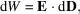where [equation (1.1.3.3)]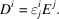Since both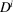and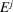are expressed through contravariant components, the expression for the energy should be written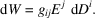If we replaceby its expression, we obtain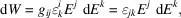where we have introduced the doubly covariant form of the dieletric constant tensor,. Differentiating twice gives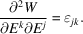If one can assume, as one usually does in physics, that the energy is a `good' function and that the order of the derivatives is of little importance, then one can write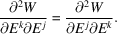As one can exchange the role of the dummy indices, one has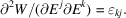Hence one deduces that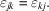The dielectric constant tensor is therefore symmetric. One notes that the symmetry is conveyed on two indices of the same variance. One could show in a similar way that the tensor representing magnetic susceptibility is symmetric. (3) There are other possible causes for the symmetry of a tensor of rank 2. The strain tensor (Section 1.3.1), which is a field tensor, is symmetric because one does not take into account the rotative part of the deformation; the stress tensor, also a field tensor (Section 1.3.1), is symmetric because one neglects body torques (couples per unit volume); the thermal conductivity tensor is symmetric because circulating flows do not produce any detectable effects etc.

#### 1.1.4.5.2.2. Tensors of higher rank

| top | pdf |

A tensor of rank higher than 2 may be symmetric with respect to the indices of one or more couples of indices. For instance, by its very nature, the demonstration given in Section 1.1.1.4shows that the tensors representing principal physical properties are of even rank. If n is the rank of the associated square matrix, the number of independent components is equal to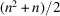. In the case of a tensor of rank 4, such as the tensor of elastic constants relating the strain and stress tensors (Section 1.3.3.2.1), the number of components of the tensor is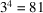. The associated matrix is a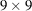one, and the number of independent components is equal to 45.

| top | pdf |

#### 1.1.4.5.3.1. Tensors of rank 2

| top | pdf |

A bilinear form is said to be antisymmetric if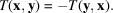Its components satisfy the relations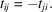The associated matrix, T, is therefore also antisymmetric: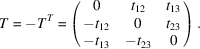The number of independent components is equal to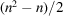, where n is the number of dimensions of the space. It is equal to 3 in a three-dimensional space, and one can consider these components as those of a pseudovector or axial vector. It must never be forgotten that under a change of basis the components of an axial vector transform like those of a tensor of rank 2.

Every tensor can be decomposed into the sum of two tensors, one symmetric and the other one antisymmetric: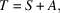with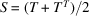and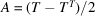.

Example. As shown in Section 1.1.3.7.2, the components of the vector product of two vectors, x and y,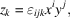are really the independent components of an antisymmetric tensor of rank 2. The magnetic quantities, B, H (Section 1.1.4.3.2), the tensor representing the pyromagnetic effect (Section 1.1.1.3) etc. are axial tensors.

#### 1.1.4.5.3.2. Tensors of higher rank

| top | pdf |

If the rank of the tensor is higher than 2, the tensor may be antisymmetric with respect to the indices of one or several couples of indices.

 (i) Tensors of rank 3 antisymmetric with respect to every couple of indices. A trilinear form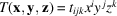is said to be antisymmetric if it satifies the relations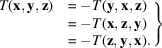Tensor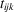has 27 components. It is found that all of them are equal to zero, except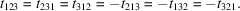The three-times contracted product with the permutations tensor (Section 1.1.3.7.2),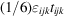, is a pseudoscalar or axial scalar. It is not a usual scalar: the sign of this product changes when one changes the hand of the reference axes, change of basis represented by the matrix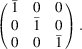Form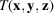can also be written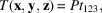where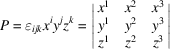is the triple scalar product of the three vectors x, y, z: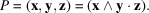It is also a pseudoscalar. The permutation tensor is not a real tensor of rank 3: if the hand of the axes is changed, the sign of P also changes; P is therefore not a trilinear form. Another example of a pseudoscalar is given by the rotatory power of an optically active medium, which is expressed through the relation (see Section 1.6.5.4)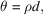where θ is the rotation angle of the light wave, d the distance traversed in the material and ρ is a pseudoscalar: if one takes the mirror image of this medium, the sign of the rotation of the light wave also changes. (ii) Tensor of rank 3 antisymmetric with respect to one couple of indices. Let us consider a trilinear form such that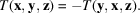Its components satisfy the relation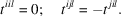The twice contracted product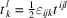is an axial tensor of rank 2 whose components are the independent components of the antisymmetric tensor of rank 3,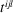.

#### Examples

 (1) Hall constant. The Hall effect is observed in semiconductors. If one takes a semiconductor crystal and applies a magnetic induction B and at the same time imposes a current density j at right angles to it, one observes an electric field E at right angles to the other two fields (see Section 1.8.3.4). The expression for the field can be written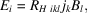where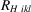is the Hall constant, which is a tensor of rank 3. However, because the direction of the current density is imposed by the physical law (the set of vectors B, j, E constitutes a right-handed frame), one has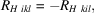which shows thatis an antisymmetric (axial) tensor of rank 3. As can be seen from its physical properties, only the components such that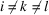are different from zero. These are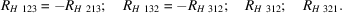(2) Optical rotation. The gyration tensor used to describe the property of optical rotation presented by gyrotropic materials (see Section 1.6.5.4) is an axial tensor of rank 2, which is actually an antisymmetric tensor of rank 3. (3) Acoustic activity. The acoustic gyrotropic tensor describes the rotation of the polarization plane of a transverse acoustic wave propagating along the acoustic axis (see for instance Kumaraswamy & Krishnamurthy, 1980). The elastic constants may be expanded as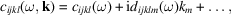where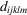is a fifth-rank tensor. Time-reversal invariance requires that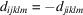, which shows that it is an antisymmetric (axial) tensor.

#### 1.1.4.5.3.3. Properties of axial tensors

| top | pdf |

The two preceding sections have shown examples of axial tensors of ranks 0 (pseudoscalar), 1 (pseudovector) and 2. They have in common that all their components change sign when the sign of the basis is changed, and this can be taken as the definition of an axial tensor. Their components are the components of an antisymmetric tensor of higher rank. It is important to bear in mind that in order to obtain their behaviour in a change of basis, one should first determine the behaviour of the components of this antisymmetric tensor.

### References

Kumaraswamy, K. & Krishnamurthy, N. (1980). The acoustic gyrotropic tensor in crystals. Acta Cryst. A36, 760–762.